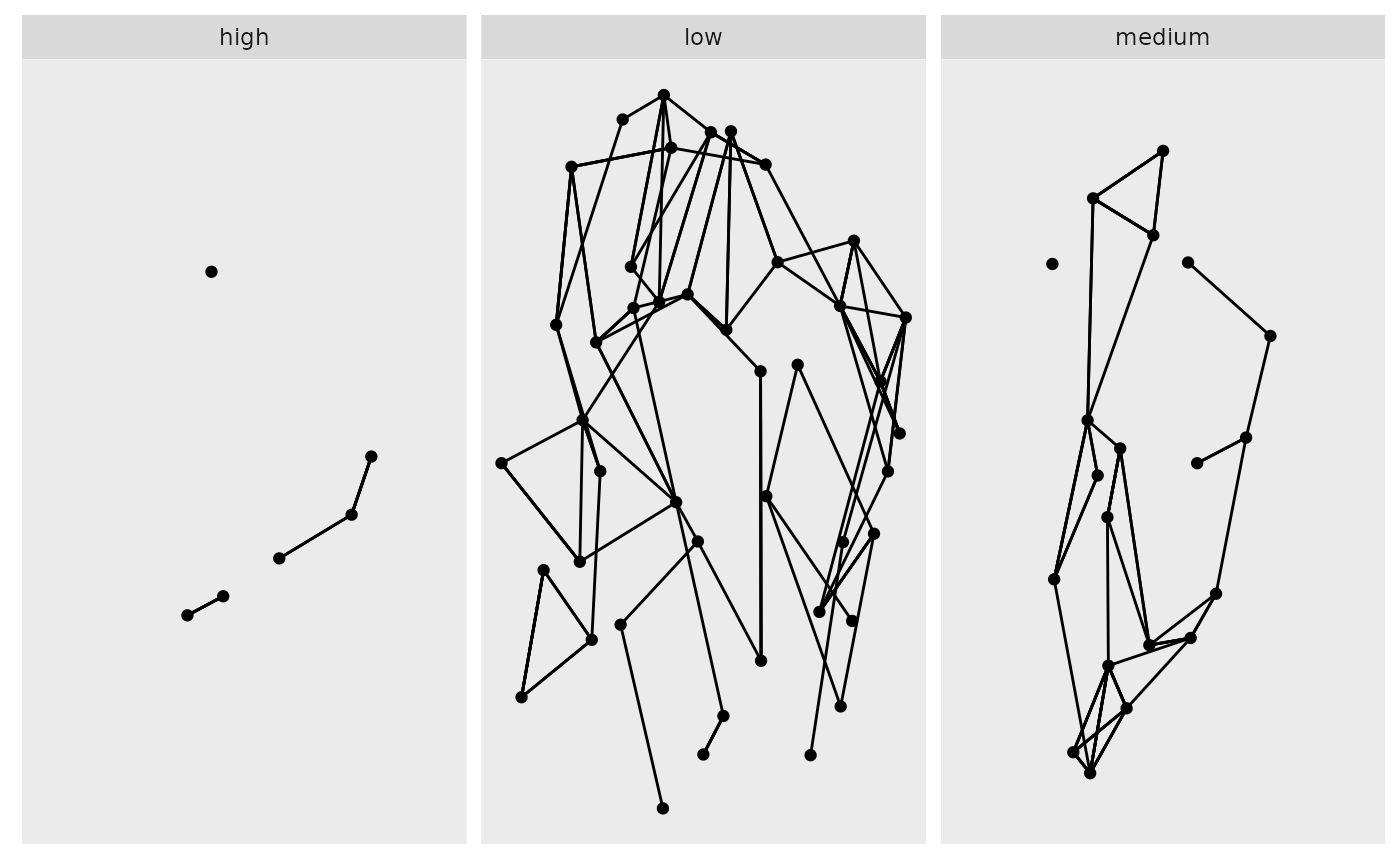This function is equivalent to ggplot2::facet_wrap() but only facets nodes. Edges are drawn if their terminal nodes are both present in a panel.

facet_nodes(
facets,
nrow = NULL,
ncol = NULL,
scales = "fixed",
shrink = TRUE,
labeller = "label_value",
as.table = TRUE,
switch = NULL,
drop = TRUE,
dir = "h",
strip.position = "top"
)

## Arguments

facets A set of variables or expressions quoted by vars() and defining faceting groups on the rows or columns dimension. The variables can be named (the names are passed to labeller). For compatibility with the classic interface, can also be a formula or character vector. Use either a one sided formula, ~a + b, or a character vector, c("a", "b"). Number of rows and columns. Number of rows and columns. Should scales be fixed ("fixed", the default), free ("free"), or free in one dimension ("free_x", "free_y")? If TRUE, will shrink scales to fit output of statistics, not raw data. If FALSE, will be range of raw data before statistical summary. A function that takes one data frame of labels and returns a list or data frame of character vectors. Each input column corresponds to one factor. Thus there will be more than one with vars(cyl, am). Each output column gets displayed as one separate line in the strip label. This function should inherit from the "labeller" S3 class for compatibility with labeller(). You can use different labeling functions for different kind of labels, for example use label_parsed() for formatting facet labels. label_value() is used by default, check it for more details and pointers to other options. If TRUE, the default, the facets are laid out like a table with highest values at the bottom-right. If FALSE, the facets are laid out like a plot with the highest value at the top-right. By default, the labels are displayed on the top and right of the plot. If "x", the top labels will be displayed to the bottom. If "y", the right-hand side labels will be displayed to the left. Can also be set to "both". If TRUE, the default, all factor levels not used in the data will automatically be dropped. If FALSE, all factor levels will be shown, regardless of whether or not they appear in the data. Direction: either "h" for horizontal, the default, or "v", for vertical. By default, the labels are displayed on the top of the plot. Using strip.position it is possible to place the labels on either of the four sides by setting strip.position = c("top", "bottom", "left", "right")

Other ggraph-facets: facet_edges(), facet_graph()

## Examples

library(tidygraph)
gr <- as_tbl_graph(highschool) %>%
mutate(popularity = as.character(cut(centrality_degree(mode = 'in'),
breaks = 3,
labels = c('low', 'medium', 'high')
)))
ggraph(gr) +
facet_nodes(~popularity)#> Using stress as default layout